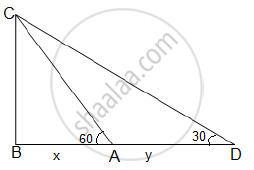Share

# The angle of elevation of an aeroplane from point A on the ground is 60˚. After flight of 15 seconds, the angle of elevation changes to 30˚. If the aeroplane is flying at a constant height of 1500√3 m, find the speed of the plane in km/hr. - CBSE Class 10 - Mathematics

#### Question

The angle of elevation of an aeroplane from point A on the ground is 60˚. After flight of 15 seconds, the angle of elevation changes to 30˚. If the aeroplane is flying at a constant height of 1500√3 m, find the speed of the plane in km/hr.

#### Solution

Let BC be the height at which the aeroplane is observed from point A.
Then, BC = 1500√3
In 15 seconds, the aeroplane moves from point A to D.
A and D are the points where the angles of elevation 60 and 30
are formed respectively.
Let BA = x metres and AD y metres
BC = x + yIn ΔCBA,

tan60^@="BC"/"BA"

sqrt3=(1500sqrt3)/x

x=1500 m........(1)

In ΔCBD,

tan30^@="BC"/"BD"

1/sqrt3=(1500sqrt3)/(x+y)

x + y =1500(3)= 4500
1500 + y = 4500
y = 3000 m ....(2)

We know that the aeroplane moves from point A to D in 15 seconds and the distance covered is 3000 metres. (by 2)

"speed"="distance"/"time"

"speed"=3000/15

speed=200 m/s

Converting it to km/hr =200x(18/5)=720 km/hr

Is there an error in this question or solution?

#### Video TutorialsVIEW ALL 

Solution The angle of elevation of an aeroplane from point A on the ground is 60˚. After flight of 15 seconds, the angle of elevation changes to 30˚. If the aeroplane is flying at a constant height of 1500√3 m, find the speed of the plane in km/hr. Concept: Heights and Distances.
S